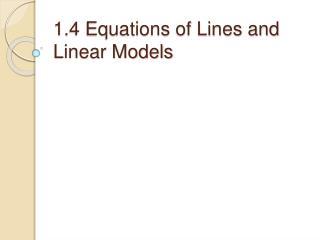Download Presentation1.4 Equations of Lines and Linear Models1.4 Equations of Lines and Linear Models - PowerPoint PPT Presentation

Download Presentation1.4 Equations of Lines and Linear Models
An Image/Link below is provided (as is) to download presentation

Download Policy: Content on the Website is provided to you AS IS for your information and personal use and may not be sold / licensed / shared on other websites without getting consent from its author. While downloading, if for some reason you are not able to download a presentation, the publisher may have deleted the file from their server.

- - - - - - - - - - - - - - - - - - - - - - - - - - - E N D - - - - - - - - - - - - - - - - - - - - - - - - - - -
Presentation Transcript

1. 1.4 Equations of Lines and Linear Models

2. Quiz • If two distinct lines, y=m1x+b1, y=m2x+b2 , are parallel with each other, what’s the relationship between m1 and m2?

3. Point-Slope Form Given the slope m of a linear function and a point (x1,y1) on the graph of the linear function. We write the equation of the linear function as y-y1=m(x-x1) We call a linear equation in this form as point-slope form of a linear function.

4. Standard Form A linear equation written in the form Ax+By=C, where A,B, and C are real numbers(A and B not both 0), is said to be in standard form. Notice: When A≠0, B=0, the linear equation will be Ax=C, which is a vertical line and is not a linear function.

5. Point-Slope Form Exercise: Write the equation of the line through (-1,3) and (-2,-3). Does it matter which point is used?

6. Parallel and Perpendicular Lines Parallel Lines: Two distinct non-vertical lines are parallel if and only if they have the same slope. y=m2x+b2 y y=m1x+b1 m1=m2 x

7. Parallel and Perpendicular Lines Perpendicular Lines: Two lines, neither of which is vertical, are perpendicular if and only if their slopes have product -1 y y=m2x+b2 y=m1x+b1 m1 × m2=-1 x

8. Parallel and Perpendicular Lines Exercises: 1, Write the equation of the line through (-4,5) that is parallel to y=(1/2)x+4 2, Write the equation of the line through (5,-1) that is perpendicular to 3x-y=8. Graph both lines by hand and by using the GC. 3, Write the equation of the line through (2/3,-3/4) that is perpendicular to y=1. Graph both lines by hand and by using the GC.

9. Linear Applications Example 1: The cellular Connection charges \$60 for a phone and \$29 per month under its economy plan, Write an equation that can model the total cost, C, of operating a Cellular Connection phone for t months. Find the total cost for six months.

10. Linear Application Example 2: The number of land-line phones in the US has decreased from 101 million in 2001 to 172 million in 2006. What is the average rate of change for the number of land-line phones over that time? Predict how many land-line phones are in use in 2010.

11. Linear Regression Why Linear Regression? In most real-life situations data seldom fall into a precise line. Because of measurement errors or other random factors, a scatter plot of real-world data may appear to lie more or less on a line, but not exactly. Fitting lines to data is one of the most important tools available to researchers who need to analyze numerical data.

12. Linear Regression • http://www.youtube.com/watch?v=nw6GOUtC2jY&feature=related

13. Linear Regression Example 1: US infant mortality 1, Find the regression line for the infant mortality data. 2, Estimate the infant mortality rate in 1995. 3, Predict mortality rate in 2006.

14. Homework • PG. 42: 5-60(M5); 61, 63, 65, Supplement(linear Regression) • KEY: 20, 45, 50, 63, S: 3, 5 • Reading: 1.5 Solving Equation & Inequalities